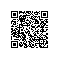# HashMap分析及散列的冲突处理

1，Hashing过程

 1 final int hash(Object k) {
2         int h = hashSeed;
3         if (0 != h && k instanceof String) {
4             return sun.misc.Hashing.stringHash32((String) k);
5         }
6
7         h ^= k.hashCode();
8         h ^= (h >>> 20) ^ (h >>> 12);
9         return h ^ (h >>> 7) ^ (h >>> 4);
10     }

static int indexFor(int h, int length) {
// assert Integer.bitCount(length) == 1 : "length must be a non-zero power of 2";
return h & (length-1);
}

indexFor(int h, int length)实际上完成的就是求余操作。只不过求余操作涉及到除法，而这里可以通过移位操作来代替除法。即二者完成的功能都是一样的，移位的效率更高。

T value = hashmap.get(key)

①get(key)时，一步计算出该key所对应的底层数组array的 index  (相当于上面 hash(Object k ) 和 indexFor(int h, int length) 这两个函数完成的功能)

②value = array[index]

2，冲突处理

public class HashMap<K,V>
extends AbstractMap<K,V>
implements Map<K,V>, Cloneable, Serializable
{
static final Entry<?,?>[] EMPTY_TABLE = {};
.....
.....

HashMap中有一个Entry数组，而Entry类是HashMap的内部类。由Entry类来封装实际的<Key, Value>

 static class Entry<K,V> implements Map.Entry<K,V> {
final K key;
V value;
Entry<K,V> next;
int hash;

HashMap中还有两个变量： int threshold 和 float loadFactor。loadFactor 默认是0.75，threshold作用如下：当HashMap中的元素个数超过threshold时，就会重新调整哈希的大小。

void addEntry(int hash, K key, V value, int bucketIndex) {
if ((size >= threshold) && (null != table[bucketIndex])) {
resize(2 * table.length);

 threshold = (int)Math.min(newCapacity * loadFactor, MAXIMUM_CAPACITY + 1);使用钉钉扫一扫加入圈子
+ 订阅